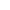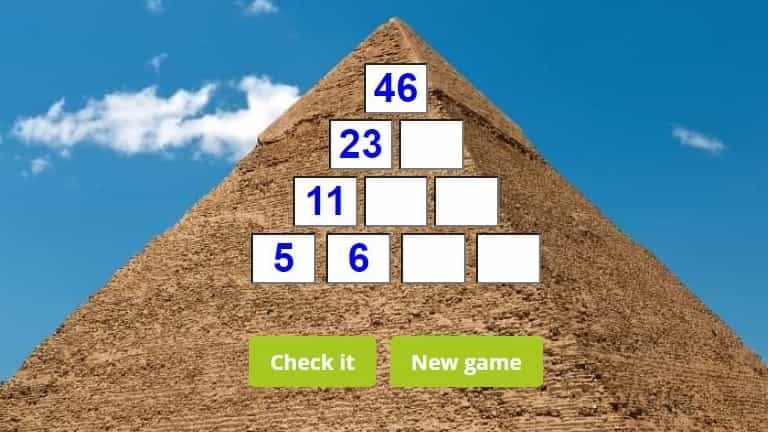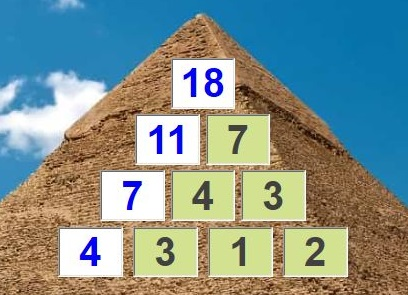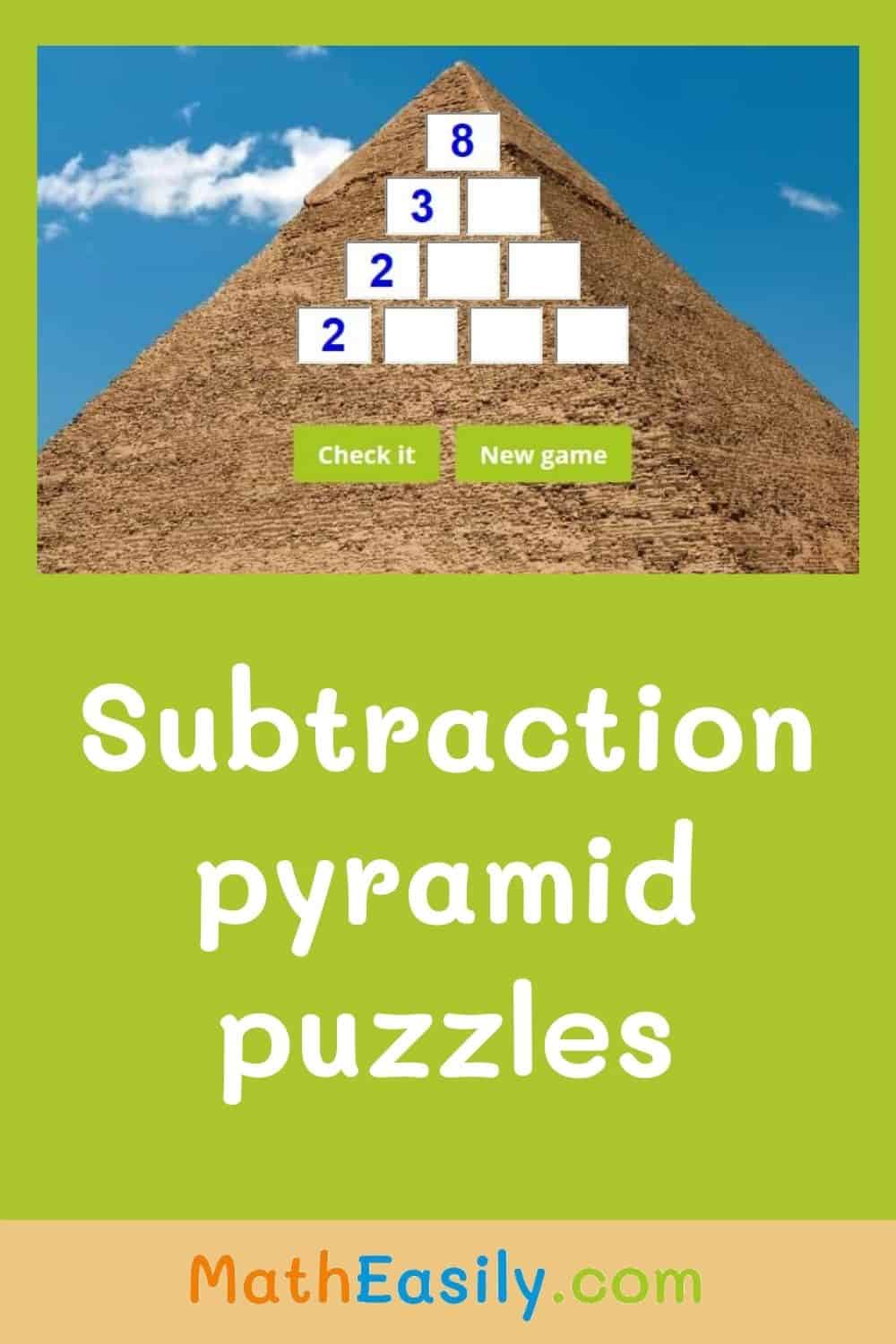﻿ Subtraction pyramid puzzle | worksheets and games

Page No. 1087

# Subtraction pyramids: worksheets and games

Subtraction pyramid puzzle game. Subtraction puzzles online. Addition and subtraction pyramids. Subtraction puzzle worksheets. missing number pyramid puzzle.

New gameFill in missing numbers in this subtraction pyramid.
Click the blue button to choose the maximum value on the top: 20, 30, 50 or 100:

## Subtraction pyramid gamesSubtraction pyramids not only serve to teach subtraction but they also develop logical thinking. First, kids have to find the right place where to start with solving of these subtraction pyramid games. The subtraction pyramid puzzle should be solved from the left to the right side.

## How to solve subtraction pyramid puzzle?

In subtraction pyramids, numbers next to each other are added together to make the number above it.

Insert the missing numbers to the empty boxes of the subtraction pyramid puzzle. Here is the sample of the right solved subtraction pyramid puzzle:Press "Check it" to find out if your answer is OK. The correct boxes will be green, wrong boxes will be red. Press the button "New game" and the new subtraction pyramid puzzle will be generated.

## Subtraction pyramid worksheets

In these subtraction pyramid puzzles kids must subtract the given number from the number above it, so the result of the adittion of two neighboring numbers equals the number above them.

Our free printable subtraction pyramid worksheets in PDF are generated automatically. Each addition and subtraction pyramid worksheet consists of two pages: the first page is the worksheet, the other page contains the answer key. You can download more math subtraction worksheets here.

## Subtraction pyramids puzzle

Subtraction pyramid game is a nice way how to learn subtraction through games. Maximum value which can occur in this subtraction pyramid puzzle is up to you. You can choose: 20, 30, 50 or 100.

To play this subtraction pyramid puzzle game kids need to have a good knowledge of single digit subtraction and subtraction within 20 and 2 digit subtraction.

Do you want to play addition pyramid game? Then try this addition pyramid puzzle.

### You might also like these number pyramid games: